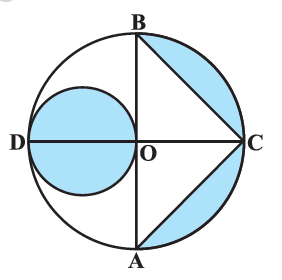# Ex.12.3 Q9 Areas Related to Circles Solution - NCERT Maths Class 10

Go back to  'Ex.12.3'

## Question

In Figure, $$AB$$ and $$CD$$ are two diameters of a circle (with center $$O$$) perpendicular to each other and $$OD$$  is the diameter of the smaller circle. If $$OA = \rm{7 cm,}$$ find the area of the shaded region.Video Solution
Areas Related To Circles
Ex 12.3 | Question 9

## Text Solution

What is known?

$$AB$$ and $$CD$$ are two diameters of a circle with centre $$O,$$ perpendicular to each other that is $$\,\angle {BOC} = {90^ \circ }$$

$$OD$$ is diameter of smaller circle,and $$OA = \text{7 cm.}$$

What is unknown?

Reasoning:

Since $$AB$$ and $$CD$$ are diameter of the circle.

\begin{align}\therefore \quad OD&= OC = OA=OB=R=7\rm{}cm\end{align} (being radii of the circle)

$$\therefore$$  $$\rm{}AB=CD=2R=14\, cm$$

$$\therefore$$  Radius of shaded smaller circular region \begin{align}r = \frac{{OD}}{2} = \frac{7}{2}\rm{cm} \end{align}

Area of the shaded smaller circular region \begin{align} = \pi {\left( {\frac{7}{2}} \right)^2}\end{align}

Area of shaded segment of larger circular region $$=$$ Area of semicircle $$ACB$$ $$–$$  Area of $$\Delta {ABC}$$

\begin{align} &= \frac{\pi }{2}{{(OA)}^2} - \frac{1}{2}\!\times {AB}\!\times\!{OC}\\&\qquad\left( {\therefore \angle BOC = {{90}^\circ }} \right)\\ &= \frac{\pi }{2}{7^2} - \frac{1}{2} \times 14 \times 7\AB &= OA + OB = 20A = 14)\end{align} Area of shaded region \(= Area of shaded smaller cricular region $$\rm{} \,+$$ Area of shaded sagment of larger circular region

Steps:

$$\text{OA = 7 cm}$$

$$AB$$ and $$CD$$ are diameter of the circle with center $$O$$

\begin{align}&\therefore OD = OC = OA = OB = R = 7cm\end{align}(being radii of the circle)

\begin{align} \therefore AB = 2R = 14cm\end{align}

Radius of shaded circular region $$= \frac{{OD}}{2} = \frac{7}{2}{\text{cm}}$$

Area of shaded smaller circular region \begin{align}= \pi {r^2}\end{align}

\begin{align}&= \pi {\left( {\frac{7}{2}cm} \right)^2}\\&= \frac{{22}}{7} \times \frac{7}{2} \times \frac{7}{2}c{m^2}\\&= \frac{{77}}{2}c{m^2}\\&= 38.5c{m^2}\end{align}

Area of shaded segment of larger circular region $$=$$  Area of semicircle $$ACB\; -$$ Area of  $$\Delta {ABC}$$

\begin{align} & =\left[ \begin{array} & \frac{1}{2}\pi {{\left( OA \right)}^{2}}- \\ \frac{1}{2}\times AB\times OC \\ \end{array} \right] \\ & \left( \therefore OC\bot AB \right) \\ & =\frac{1}{2}\pi {{R}^{2}}-\frac{1}{2}\times 2R\times R \\ & =\left[ \begin{array} & \frac{1}{2}\times \frac{22}{7}\times {{\left( 7cm \right)}^{2}}- \\ \frac{1}{2}\times 14cm\times 7cm \\ \end{array} \right] \\ & =77c{{m}^{2}}-49c{{m}^{2}} \\ & =28c{{m}^{2}} \\ \end{align}

Area of shaded region = Area of the shaded smaller circular region + Area of the shaded segment of larger circular region.

\begin{align}&= 38.5c{m^2} + 28c{m^2}\\&= 66.5c{m^2}\end{align}

Learn from the best math teachers and top your exams

• Live one on one classroom and doubt clearing
• Practice worksheets in and after class for conceptual clarity
• Personalized curriculum to keep up with school